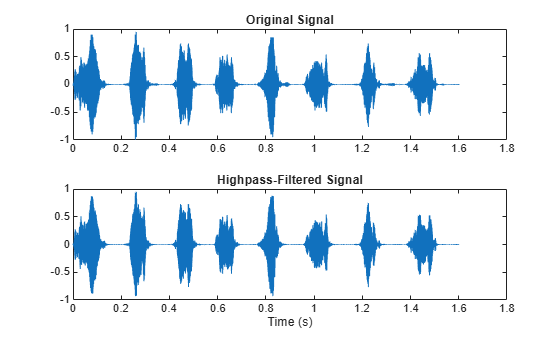# sosfilt

## Syntax

``y = sosfilt(sos,x)``
``y = sosfilt(sos,x,dim)``

## Description

example

````y = sosfilt(sos,x)` applies the second-order section digital filter `sos` to the input signal `x`.```
````y = sosfilt(sos,x,dim)` operates along the dimension `dim`.```

## Examples

collapse all

Load `chirp.mat`. The file contains a signal, `y`, that has most of its power above `Fs/4`, or half the Nyquist frequency. The sample rate is 8192 Hz.

```load chirp t = (0:length(y)-1)/Fs;```

Design a seventh-order Butterworth highpass filter to attenuate the components of the signal below `Fs/4`. Use a normalized cutoff frequency of 0.48π rad/sample. Express the filter coefficients in terms of second-order sections.

```[zhi,phi,khi] = butter(7,0.48,'high'); soshi = zp2sos(zhi,phi,khi); freqz(soshi)```Filter the signal. Display the original and highpass-filtered signals. Use the same y-axis scale for both plots.

```outhi = sosfilt(soshi,y); figure subplot(2,1,1) plot(t,y) title('Original Signal') ys = ylim; subplot(2,1,2) plot(t,outhi) title('Highpass-Filtered Signal') xlabel('Time (s)') ylim(ys)```Design a lowpass filter with the same specifications. Filter the signal and compare the result to the original. Use the same y-axis scale for both plots. The result is mostly noise.

```[zlo,plo,klo] = butter(7,0.48); soslo = zp2sos(zlo,plo,klo); outlo = sosfilt(soslo,y); subplot(2,1,1) plot(t,y) title('Original Signal') ys = ylim; subplot(2,1,2) plot(t,outlo) title('Lowpass-Filtered Signal') xlabel('Time (s)') ylim(ys)```## Input Arguments

collapse all

Second-order section digital filter, specified as an L-by-6 matrix, where L is the number of second-order sections. The matrix

`$\text{sos}=\left[\begin{array}{cccccc}{b}_{01}& {b}_{11}& {b}_{21}& 1& {a}_{11}& {a}_{21}\\ {b}_{02}& {b}_{12}& {b}_{22}& 1& {a}_{12}& {a}_{22}\\ ⋮& ⋮& ⋮& ⋮& ⋮& ⋮\\ {b}_{0L}& {b}_{1L}& {b}_{2L}& 1& {a}_{1L}& {a}_{2L}\end{array}\right]$`

represents the second-order section digital filter

`$H\left(z\right)=\prod _{k=1}^{L}{H}_{k}\left(z\right)=\prod _{k=1}^{L}\frac{{b}_{0k}+{b}_{1k}{z}^{-1}+{b}_{2k}{z}^{-2}}{1+{a}_{1k}{z}^{-1}+{a}_{2k}{z}^{-2}}.$`

Example: `[b,a] = butter(3,1/32); sos = tf2sos(b,a)` specifies a third-order Butterworth filter with a normalized 3 dB frequency of π/32 rad/sample.

Data Types: `single` | `double`

Input signal, specified as a vector, matrix, or N-D array.

Example: `x = [2 1].*sin(2*pi*(0:127)'./[16 64])` specifies a two-channel sinusoid.

Data Types: `single` | `double`
Complex Number Support: Yes

Dimension to operate along, specified as a positive integer scalar. By default, `sosfilt` operates along the first array dimension of `x` with size greater than 1.

Data Types: `single` | `double`

## Output Arguments

collapse all

Filtered signal, returned as a vector, matrix, or N-D array. `y` has the same size as `x`.

 Bank, Balázs. "Converting Infinite Impulse Response Filters to Parallel Form". IEEE Signal Processing Magazine. Vol. 35, Number 3, May 2018, pp. 124-130.

 Orfanidis, Sophocles J. Introduction to Signal Processing. Englewood Cliffs, NJ: Prentice-Hall, 1996.